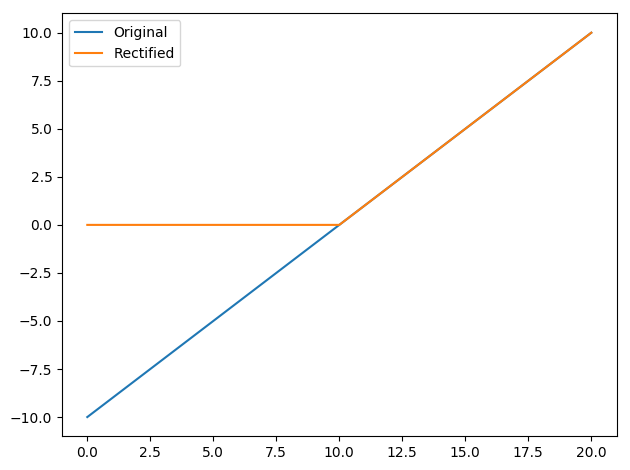Fixtures for quickly making Matplotlib plots in tests

## pytest-plt

pytest-plt provides fixtures for quickly creating Matplotlib plots in your tests.

Create PDF plots in one line with the plt fixture.

def test_rectification(plt):
values = list(range(-10, 11))
rectified = [v if v > 0 else 0 for v in values]
assert all(v >= 0 for v in rectified)
plt.plot(values, label="Original")
plt.plot(rectified, label="Rectified")
plt.legend()To use these fixtures, install with

pip install pytest-plt

And pass the --plots option

pytest --plots

### Usage

The plt fixture allows you to create PDF plots with as little as one line. It exposes the matplotlib.pyplot interface.

When running your tests, pass the --plots option (with optional directory name) to generate the plots (in this case, we save them to the “my_plots” directory):

pytest --plots my_plots

If no directory name is provided, plots will be saved to the “plots” directory:

pytest --plots

If you do not pass the --plots option, no Matplotlib commands will be executed, speeding up test execution.

#### Custom filenames and extensions

pytest-plt attempts to give each plot a readable name without being too long. Sometimes the default name is not good enough, so plt allows you to change it using plt.saveas:

def test_rectification(plt):
values = list(range(-10, 11))
rectified = [v if v > 0 else 0 for v in values]
assert all(v >= 0 for v in rectified)
plt.plot(values, label="Original")
plt.plot(rectified, label="Rectified")
plt.legend()
plt.saveas = "test_rec.png"

The plt.saveas attribute contains the filename that will appear in the plots directory. You can modify this attribute within your test to change the filename that will be used to save the plot for a given test function. In the above example, running pytest with pytest --plots my_plots will result in a my_plots/test_rec.png file.

It should be noted that the file extension in plt.saveas will be used when saving the plot. That is, in the example above, the resulting plot will be a true PNG file, not a PDF with an incorrect .png extension. You can use the following snippet to change the file extension in a specific test if the PDF format is unsuitable.

plt.saveas = "%s.png" % (plt.saveas[:-4],)

#### Using plt.show

If you want to inspect a figure using the GUI that pops up with plt.show(), you can achieve this by saving a plot with the .pkl or .pickle extension. pytest-plt will pickle the current figure object for later inspection.

Building on the previous example, you can change the saveas attribute like so:

def test_rectification(plt):
values = list(range(-10, 11))
rectified = [v if v > 0 else 0 for v in values]
assert all(v >= 0 for v in rectified)
plt.plot(values, label="Original")
plt.plot(rectified, label="Rectified")
plt.legend()
plt.saveas = "test_rec.pkl"

Then use the following snippet to inspect the figure.

>>> import pickle
>>> import matplotlib.pyplot as plt
>>> with open("path/to/test_rec.pkl", "rb") as fh:
>>> plt.show()

### Configuration

The following configuration options exist.

#### plt_filename_drop

plt_filename_drop accepts a list of regular expressions for parts of the filename to drop.

By default, plot filenames contain the full nodeid for the test in question, with directory separators (/) replaced with dots (.). If all tests reside in the same project directory, that name will appear at the start of all plot filenames, making them unnecessarily long.

In this case, we use the carat ^ to ensure that our regex matches the start of the filename only, and we remove the trailing dot as well (\.):

plt_filename_drop =
^project\.

If your tests always reside in a directory with a particular name (e.g. “tests”), you can safely remove this name wherever it occurs. In this case, we do not use the carat to allow the regex to match anywhere in the filename. Be careful, as this will match any directory that ends with “tests” (e.g. “other_tests”), and will remove the ends of these directory names.

plt_filename_drop =
^project\.
tests\.

When using plt_filename_drop, take care to avoid collisions (situations where plots from two different tests will end up with the same name). In this case, the plots of later tests will override those of earlier tests with the same name.

#### plt_dirname

plt_dirname changes the default directory name for output plots.

The default plt_dirname is "plots". To change it to "test_plots", for example, add the following to your pytest.ini.

plt_dirname = test_plots

A directory provided at the command line with the --plots flag takes priority over plt_dirname.

See the full documentation for more details and configuration options.

## Release History

### 1.1.0 (August 17, 2020)

• Added the ability to save plots as pickle files using the .pkl or .pickle extensions with plt.saveas. (#23)

### 1.0.1 (October 28, 2019)

Fixed

• We now use Windows-compatible plot filenames by default. Colons in plot filenames are replaced with hyphens. Filenames specified through plt.saveas are not modified. (#17, #21)

### 1.0.0 (August 9, 2019)

Initial release of pytest-plt! Thanks to all of the contributors for making this possible!

## Project details

This version1.1.01.0.11.0.0

Uploaded source
Uploaded py3